﻿ Influence of the Doping Rate of the CIGSe Layer on the Recombination-generation Mechanisms with 25 nm Incorporation of a KF Layer on a CIGSe Solar CellPublications are Open
Access in this journal
Article Versions
Export Article
• Normal Style
• MLA Style
• APA Style
• Chicago Style
Research Article
Open Access Peer-reviewed

### Influence of the Doping Rate of the CIGSe Layer on the Recombination-generation Mechanisms with 25 nm Incorporation of a KF Layer on a CIGSe Solar Cell

Djimba Niane , Alain K. Ehemba, Salif Cissé, Ousmane Diagne, Moustapha Dieng
American Journal of Energy Research. 2018, 6(1), 30-34. DOI: 10.12691/ajer-6-1-5
Published online: July 19, 2018

### Abstract

In this work, we studied the influence of the doping rate of the CIGSe layer on the recombination-generation mechanisms with 25 nm incorporation of a KF layer on a CIGSe solar cell. The different doping taken are Nb=1015cm-3, Nb=1016cm-3 et Nb=1017cm-3. Numerical modeling with the SCAPS-1D software shows that the generation rate varies slightly while that of recombination is very important, explained by a strong presence of traps in the CIGSe bandgap.

### 1. Introduction

The history of the technology of solar cell based copper gallium indium and diselenium (CIGSe) solar cell technology is a remarkable scientific and technological adventure whose progress has allowed yields to increase by 9% in the 1980 more than 22% 1 today took place by successive technological leaps. Despite these advantages, solar cells CIGS are the object of many research, because these cells have a composite structure complexes which consists of stacked layers of several materials. In this paper, we simulate the influence of the doping rate of the CIGSe layer on the generation and recombination rate of the solar cell. This study is done by depositing a 25nm layer of kF between the CIGSe and the CdS. The study is based on a n-ZnO / n-CdS / p-CIGS cell as shown in Figure 1.

• Figure 1. Structure of a heterojunction solar cell n-ZnO/n-CdS/p-CIGSe

### 2. Numerical Modelling

In the literature, carrier generation is described as the process in which electron-hole pairs are created by exciting an electron in the valence band of the semiconductor thus creating a hole in the valence band. Recombination of carriers in a semiconductor is the reverse phenomenon of generation. It corresponds to the loss mechanisms of electron-hole pairs accompanied by excess energy emitted in the form of phonons or photons. A theory describing these two phenomena has been established by Shockley, Read and Hall 2, 3. According to this theory, there are four under processes responsible for generations and for the recombination of the carriers. Electron capture followed by hole capture leads to a recombination process, whereas, hole emission followed by electron emission leads to generation of an electron-hole pair.

In this study, modeling was made with software SCAPS-1D 4, 5. In its default mode, SCAPS calculates the generation of electron-hole pairs G(x) from the incident photon flux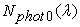from a very coarse optical model. This model contains only reflection /transmission at the two contacts, and the absorption in the layers. From the photon flux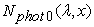at each position and for each wavelength, the generation of eh pairs G(x) is then calculated as: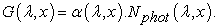(1)

This is then integrated over all wavelengths of the incoming photon flux: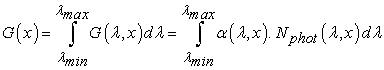(2)

Where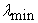and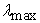are the short and long wavelength limits of the incoming photon spectrum.

The rate of recombination can be written as: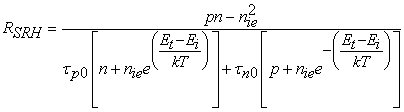(3)

Where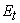is the energy level associated with the defect,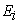is the intrinsic energy level, n, p are the concentration of the electrons and the holes respectively out of equilibrium such that n=n0 +∆n et p= p0 +∆p,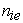the intrinsic concentration, k the Boltzmann constant, T the temperature and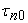,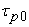the lifetime for the electrons and the holes respectively.

With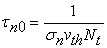(4)

and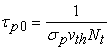(5)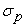,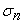are the capture cross-sections of the holes and the electrons respectively,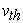is the thermal speed of the charge carriers, and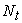is the concentration of carriers located at the level.

The properties of the bulk defects of the different layers are presented in Table 1:

### 3. Results and Discussions

3.1. Capture and Emission Rates of the Electron

The distribution function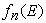represents the probability that a trap is occupied by an electron. Its expression is given by: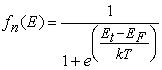(6)

3.1.1. Emission Rate of Electrons

The electron emission rate is determined by knowing the number of places available for an electron in the conduction band. It results from it that the emission of an electron takes place when the electron leaves a neutral trap to go towards the conduction band. Consequently, the emission rate of an electron is expressed as follows: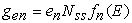(7)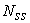is the density of traps and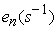is the probability of emission of an electron trapped to the conduction band.

Figure 2 illustrates the electron emission rate according of the depth of the CIGSe base for different doping of the CIGSe layer.

• Figure 2. Emission rate of electrons according of the depth of the CIGSe base for different doping of the CIGSe layer

In this figure, the electron emission rate was simulated through the SCAPS-1D software by fixing the thickness of the 25 nm KF layer. The different doping taken are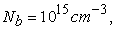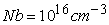and. We observe in Figure 2 that the emission rate decreases with the increase in the doping of the base. Indeed, the coefficient of the electron emission depends on the energetic position of the traps in a semiconductor. Moreover, the increase in the doping of the layer of CIGSe accentuates the density of the holes in the layer of CIGSe and consequently emptying traps increases.

3.1.2. Capture Rate of Electrons

There is capture of an electron when this one leaves the conduction band to go towards to the valence band to occupy a trap ionized earlier. The capture rate of electrons characterizes the fact that an electron that transits is not always trapped. Taking into account the capture probability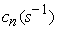, the number of electrons present in the conduction band and the number of unoccupied traps; the electron capture rate per unit volume is expressed by the following relation: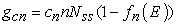(8)

With: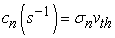.

Whererepresents the thermal velocity of the carriers andthe effective cross-section of electron capture by the traps.

Figure 3 shows the capture rate of electrons as a function of the depth of the base for different doping rates of the CIGSe layer.

• Figure 3. Capture rate of electrons as a function of the depth of the CIGSe for different doping rates of the CIGSe layer

At first, we notice that the capture rate of electrons at the base increases according to his thickness when the carriers cross the layer of CIGSe until reach a maximum. A progressive decrease of the rate of capture is noted when the carriers get closer to the back contact. In a second time, the analysis of the curve also shows that the rate of capture of electrons per unit of volume increases remarkably when the doping rate of the base increases inside the layer of CIGSe. Moreover, we notice the depth corresponding to the maximum reached by the capture rate increases as a function of the doping rate inside the base. This results in the increase of holes in the base which favors the filling of the traps.

3.2. Capture and Emission Rate of the Holes

We defined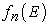as being the probability for the electron to occupy a state of energy E. Conversely, it results from it that the probability so that this state is not occupied by an electron is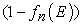. This function represents consequently the function of distribution of the holes in the semiconductor. It is expressed in the following way: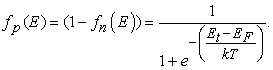(9)

3.2.1. Hole Emission Rate

The holes emission rate is equated to the number of electrons leaving the valence band to occupy earlier traps ionized. Taking into account the emission coefficient of the holes by traps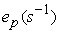, the number of empty trap door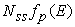, the emission rate of the holes is written: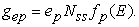(10)

The plot of the holes emission rate as a function of the depth of the base for different doping of the CIGSe layer is shown in Figure 4.

• Figure 4. Emission rate of holes as a function of the depth of the base for different doping of the CIGSe layer

Figure 4 shows that the holes emission rate increases with the doping rate. This increase is most noticeable when approaching the CIGSe/Cds interface. This particularity is explained by the fact that the increase in the emission rate observed at the level of the CIGSe layer cannot come from the increase in the number of holes which is proportional to the emission rates of the holes. Consequently, the result is a displacement of electrons hosted at the valence band to occupy centers of recombination and generations (traps).

3.2.2. Hole Capture Rate

The capture of the holes takes place when an electron occupying a trap leaves it to neutralize a hole in the valence band. The capture rate of the holes is proportional to the capture possibilities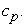Considering the concentration of free holes in the valence band p, the capture rate is expressed as follows: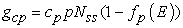(11)

With: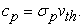is the cross section of capture of the holes by the traps.

Figure 5 shows the capture rate of the holes as a function of the depth of the base (CIGSe) for different doping of the CIGSe layer.

• Figure 5. Capture rate of the holes as a function of the depth of the base for different doping of the CIGSe layer

The remark made in Figure 5 shows a similarity of the gaits noted in Figure 3, nevertheless the capture rate of the holes is slightly greater than that of the electrons when the doping increases. Indeed, an increase in the doping of the base induces the cell in diet of accumulation, in other words a high concentration of the holes. This implies an increase in the capture rate and the probability of electron occupation at the valence band.

3.3. Carriers Generation Rate

Figure 6 shows the generation rate of the carriers for different doping of the CIGSe layer as a function of the depth of the cell.

• Figure 6. Generation rate of the carriers for different doping of the CIGSe layer as a function of the depth of the cell

The analysis of the rate of generations of the carriers per unit of volume can be done starting from the thickness division of the cell. Considering, the cell is photo-illuminated by the front contact. We can subdivide the curve of the generation rate into three parts:

When the photons arrive at the level of the cell, the first layer met is that of ZnO. In this layer corresponding to depths between 2.55 and 2.8m, we notice a decrease of the rate of generation of the carriers. This decrease is explained by the fact that in this layer, the density of the electrons is very important compared to that of the holes.

Then, for depths between 2.5 and 2.55m, we are in the CdS layer. The carrier generation rate increases in this layer. This increase is explained by the beneficial role of the buffer layer; which makes it possible, among other things, to maximize the alignment of the bands between the CIGS and the ZnO window layer, but also to favor the collection of the carriers generated in the CIGSe.

Finally, for depths between 0 and 2.5 m corresponding to the depth of the base, we note a decrease in the generation of carriers when approaching the back contact. However, a significant peak is noted when approaching the CIGSe / CdS interface. Given that the CIGSe has a direct gap, the absorption of photons with sufficient energy, at least equal to that of the gap, does not require the intervention of phonons. This results in a high optical absorption coefficient that increases the generation of electron-hole pairs in the CIGSe / CdS interface.

Besides, we notice that the rate of generations of the carriers does not vary almost with the increase of the doping of the base. However, the zoom of the figure shows that, for doping between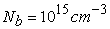and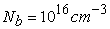the rate remains constant while for doping,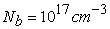, we note a net increase in the rate.

3.4. Recombination Rate of Carriers

Figure 7 shows the recombination rate of the carriers for different doping of the CIGSe layer as a function of the depth of the cell.

• Figure 7. Recombination rate of the carriers for different doping of the CIGSe layer as a function of the depth of the cell

In Figure 7, we notice that the recombination rate increases with the increase of the doping of the base. Indeed, the increase in the doping of the CIGSe layer causes disturbances that destroy the perfect periodicity of the CIGSe and cause the creation of energy states in the gap. This addition of doping atoms creates distortions in the CIGSe layer and affects carrier mobility. Consequently, this results in additional recombination of the carriers due to the increase of capture phenomena 2, 6.

### 4. Conclusion

In the p-n junctions, the generation and recombination phenomena from the traps located in the space charge zone confer the diodes electrical properties. In this work, we have shown that the doping increase of the CIGSe layer leads to very important recombination. This results the harmful effects of maximum doping in these types of solar cells. Consequently, the result is to find adequate doping so that carrier generation is maximal and recombination is minimal.

### References

  Philip Jackson , Roland Wuerz, Dimitrios Hariskos, Erwin Lotter, Wolfram Witte, and Michael Powalla, “Effects of heavy alkali elements in Cu(In,Ga)Se2solar cells with efficiencies up to 22.6%” Phys. Status Solidi RRL 10, No. 8, 583-586 (2016). In article View Article  Schockley. W, Read W.T, “Statistics of the Recombinations of Holes and Electrons” Physical Review, 1952, vol 87, n°5, p. 835-842. In article View Article  Hall R. N., “Electron-Hole Recombination in Germanium” Physical Review, 1952, vol 87 n°2 p 387. In article View Article  M. Burgelman, P. Nollet, S. Degrave, “Modelling polycrystalline semiconductor solar cells”, Thin Solid Films 361/362 (2000) pp. 527-532. In article View Article  M. Burgelman, K. Decock, S. Khelifi and A. Abass, “Advanced electrical simulation of thin film solar cells”, Thin Solid Films, 535 (2013) 296-301 In article View Article  R. C. Neville, Solar Energy Conversion: the solar cell (Elsevier, New York, 1995). In article View Article

Published with license by Science and Education Publishing, Copyright © 2018 Djimba Niane, Alain K. Ehemba, Salif Cissé, Ousmane Diagne and Moustapha DiengThis work is licensed under a Creative Commons Attribution 4.0 International License. To view a copy of this license, visit http://creativecommons.org/licenses/by/4.0/